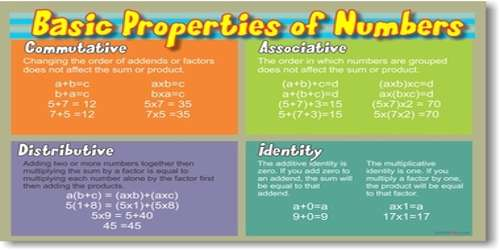There are four basic properties of numbers: commutative, associative, distributive, and identity. Basically, there are three properties. These are the commutative, associative, and the distributive property. However, we can extend them to include the properties of zero and one. We also called these properties rules of arithmetic. It is especially important to understand these properties once you reach advanced math such as algebra and calculus.

Commutative Property

• Addition: When two numbers are added, the sum is the same regardless of the order in which the numbers are added. Example: 3 + 5 = 8 or 5 + 3 = 8
• Multiplication: When two numbers are multiplied together, the product is the same regardless of the order in which the numbers are multiplied. Example: 3 x 5 = 15 or 5 x 3 = 15Associative property

The associative property of numbers is explained for both addition and multiplication. Start off with a nice real life example and at the end, see the difference between the commutative and the associative property

• Addition: When three or more numbers are added, the sum is the same regardless of the way in which the numbers are grouped. Example: 6 + (4 + 3) = 13 or (6 + 4) + 3 = 13
• Multiplication: When three or more numbers are multiplied, the product is the same regardless of the way in which the numbers are grouped. Example: 6 x (4 x 3) = 72 or (6 x 4) x 3 = 72

Distributive property

The distributive property is explained with three good examples.

The sum of two numbers times a third number is equal to the sum of each addend times the third number. Example; 5 x (7 + 2) = 45 or 5 x 7 + 5 x 2 = 45.

Identity Property

• Addition: The sum of any number and zero is that number. Example: 12 + 0 = 12
• Multiplication: The product of any number and one is that number. Example: 18 x 1 = 18

Properties of zero

Here, we explain the properties of zero. The failure to understand and/or recognize these properties may lead to some common mistakes made by students .Master these properties once and for all right here.